# FST4 Doppler Spread Algorithm in WSJT-X

At HamSCI 2023 this past weekend I was lucky enough to be able to attend Gwyn Griffiths' (G3ZIL) Identifying 14 MHz Propagation Modes Using FST4W SNR and Spectral Spread and Rob Robinett’s (AI6VN) Low Cost, High Accuracy and Stability FST4W Transmissions Using the QDX Transceiver presentations. After the presentation I was able to talk to Rob at his demo. He brought up the idea that if WSPR could calculate Doppler spread the same way FSTW4 calculates it, it might provide some useful data from people that didn’t want to start using FST4 but still wanted to run WSPR nodes. In a perfect world, it would simply be a matter of calling the same subroutine in wsprd that the jt9 uses. Unfortunately as Claude Shannon can tell you, it’s not a perfect world.

I decided to lookup how FST4 does its Doppler spread calculations and it turns out the code is all in one Fortran subroutine called dopspread:

wsjtx/lib/fst4_decode.f90
   subroutine dopspread(itone,iwave,nsps,nmax,ndown,hmod,i0,fc,fmid,w50)

! On "plotspec" special request, compute Doppler spread for a decoded signal

include 'fst4/fst4_params.f90'
complex, allocatable :: cwave(:)       !Reconstructed complex signal
complex, allocatable :: g(:)           !Channel gain, g(t) in QEX paper
real,allocatable :: ss(:)              !Computed power spectrum of g(t)
integer itone(160)                     !Tones for this message
integer*2 iwave(nmax)                  !Raw Rx data
integer hmod                           !Modulation index
data ncall/0/
save ncall

ncall=ncall+1
nfft=2*nmax
nwave=max(nmax,(NN+2)*nsps)
allocate(cwave(0:nwave-1))
allocate(g(0:nfft-1))
wave=0
fsample=12000.0
call gen_fst4wave(itone,NN,nsps,nwave,fsample,hmod,fc,1,cwave,wave)
cwave=cshift(cwave,-i0*ndown)
fac=1.0/32768
g(0:nmax-1)=fac*float(iwave)*conjg(cwave(:nmax-1))
g(nmax:)=0.
call four2a(g,nfft,1,-1,1)         !Forward c2c FFT

df=12000.0/nfft
ia=1.0/df
smax=0.
do i=-ia,ia                        !Find smax in +/- 1 Hz around 0.
j=i
if(j.lt.0) j=i+nfft
s=real(g(j))**2 + aimag(g(j))**2
smax=max(s,smax)
enddo

ia=10.1/df
allocate(ss(-ia:ia))               !Allocate space for +/- 10 Hz
sum1=0.
sum2=0.
nns=0
do i=-ia,ia
j=i
if(j.lt.0) j=i+nfft
ss(i)=(real(g(j))**2 + aimag(g(j))**2)/smax
f=i*df
if(f.ge.-4.0 .and. f.le.-2.0) then
sum1=sum1 + ss(i)                  !Power between -2 and -4 Hz
nns=nns+1
else if(f.ge.2.0 .and. f.le.4.0) then
sum2=sum2 + ss(i)                  !Power between +2 and +4 Hz
endif
enddo
avg=min(sum1/nns,sum2/nns)               !Compute avg from smaller sum

sum1=0.
do i=-ia,ia
f=i*df
if(abs(f).le.1.0) sum1=sum1 + ss(i)-avg !Power in abs(f) < 1 Hz
enddo

ia=nint(1.0/df) + 1
sum2=0.0
xi1=-999
xi2=-999
xi3=-999
sum2z=0.
do i=-ia,ia                !Find freq range that has 50% of signal power
sum2=sum2 + ss(i)-avg
if(sum2.ge.0.25*sum1 .and. xi1.eq.-999.0) then
xi1=i - 1 + (sum2-0.25*sum1)/(sum2-sum2z)
endif
if(sum2.ge.0.50*sum1 .and. xi2.eq.-999.0) then
xi2=i - 1 + (sum2-0.50*sum1)/(sum2-sum2z)
endif
if(sum2.ge.0.75*sum1) then
xi3=i - 1 + (sum2-0.75*sum1)/(sum2-sum2z)
exit
endif
sum2z=sum2
enddo
xdiff=sqrt(1.0+(xi3-xi1)**2) !Keep small values from fluctuating too widely
fmid=xi2*df                  !Frequency midpoint of signal powere

do i=-ia,ia                          !Save the spectrum for plotting
y=ncall-1
j=i+nint(xi2)
if(abs(j*df).lt.10.0) y=0.99*ss(i+nint(xi2)) + ncall-1
write(52,1010) i*df,y
1010     format(f12.6,f12.6)
enddo

return
end subroutine dopspread

So here’s the problem: wsprd is written in C and jt9 uses Fortran. It wouldn’t simply be a matter of dropping this routine somewhere at the end of wsprd’s decode loop. That being said, I think there is some value taking a deep look at how FST4 calculates Doppler spread. What follows is a deep dive into the above code.

## The Test Environment

I used the WSJT-X CMake script to clone the latest version of the modified hamlib and wsjtx and confirmed that I could compile everything. This was basically a matter of following the steps in the INSTALL file. I then downloaded 60 second FTS4 signal (210115_0058.wav) and confirmed that I could use the jt9 utility to decode it. Adding a file named plotspec in the directory where I was running it caused it to calculate the Doppler spread:

$touch plotspec$ ./jt9 -7 210115_0058.wav
0058  16  0.4 1331   CQ K9KFR EN71                              0.021
0058  -7  0.3 1101   CQ N5TM EL29                               0.054
<DecodeFinished>   0   2        0

You’ll notice there are two decodes in here, but as I work through the code I’ll only be focusing on the second one. This is because I added WRITE statements to the Fortran code to spit out representations of the variables it was using. I didn’t want to bother changing filenames for each decode, so the data is only up-to-date for the last decode performed.

## Catching the Perfect Wave

When dopspread is called, the message has already been decoded. The tones are passed to the subroutine in itone and the call gen_fst4wave subroutine is used to construct an ideal signal in cwave. Through the magic of Fortran WRITE statements, a bit of reading about Fortran formats, and some Python processing with Matplotlib, we can look at part of cwave. Here’s a small 3D plot showing the rise of the signal envelope: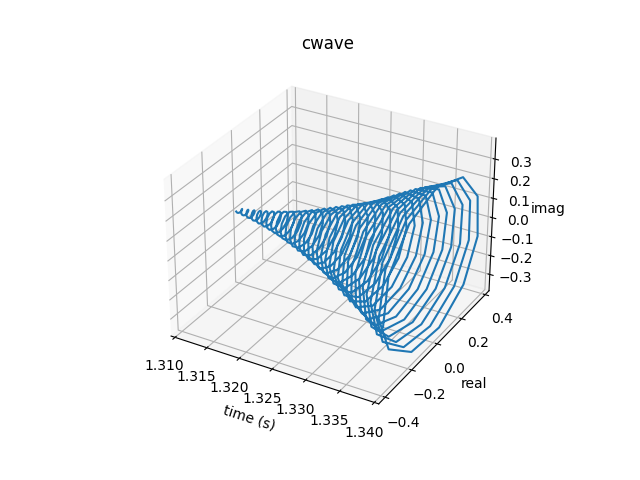Viewing it as a complex waveform doesn’t really do us too much good, so let’s look at the real projection in 2D: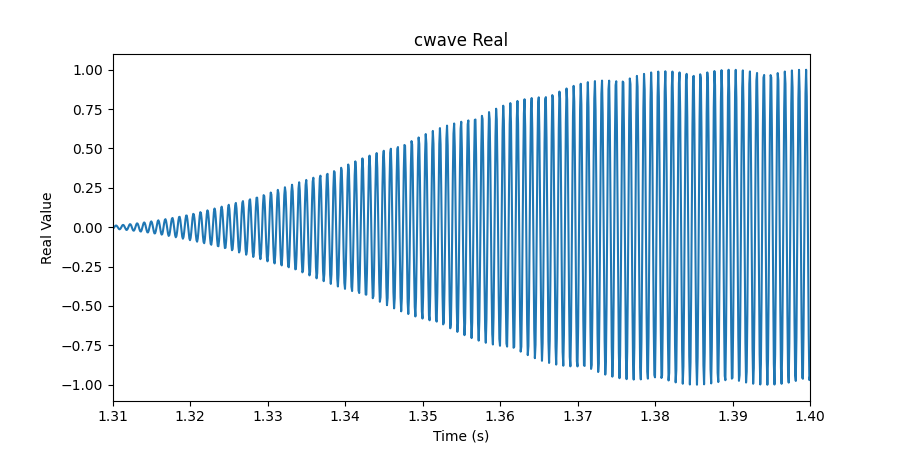Now we’re getting somewhere. You can see the Gaussian-smoothed pulses (bumps that show up when the signal increases). You’d really have to zoom in and count to actually note the minor differences in frequency, but detecting those would be no trouble for an FFT.

Remember, this waveform looks perfect because it is! We’ve already decoded this signal and this a reconstruction. Next we’ll take a look at the actual radio data that was captured.

## Riding the Wave You’re Stuck With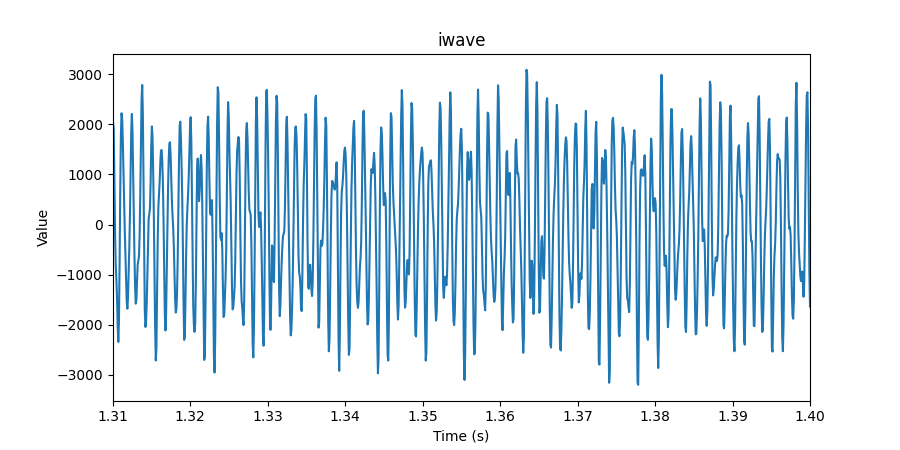This is the actual sampled RF data stored in a two byte signed integer array shown in the time domain. As you can see, it’s pretty hard for the human eye to make heads or tails of it. dopspread scales iwave down to +/- 1 and multiplies it by the complex conjugate of cwave to get g, the estimated channel gain data:

fac=1.0/32768
g(0:nmax-1)=fac*float(iwave)*conjg(cwave(:nmax-1))
g(nmax:)=0. (1)

 1 iwave starts at one and goes to nmax, but g starts at zero and goes to nmax - 1

g doesn’t help us much in the time domain, but in the frequency domain we can do a whole lot more. A complex to complex FFT with twice as many points as the samples in iwave and we get this: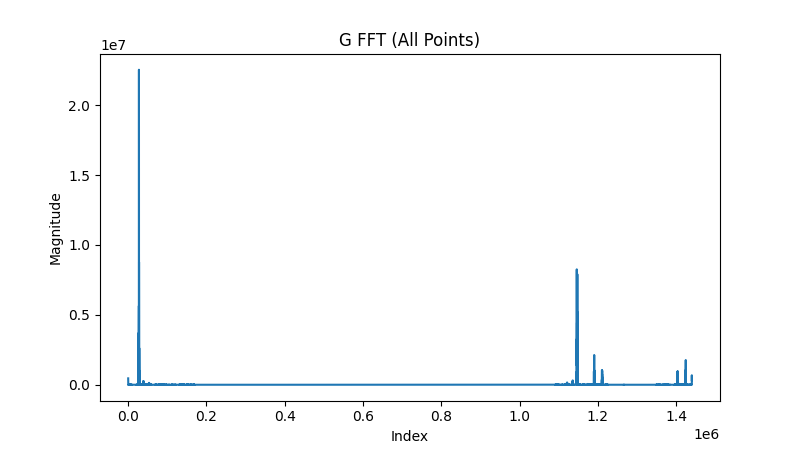This is the only FFT the algorithm performs. It’s the basis for much of the analysis that follows and it’s important to note a few things:

1. This is a representation of how much the signal has spread.

2. We only really care about the low frequencies (see the note about using a low pass filter on g in the QEX article).

3. If our signal is centered at zero, negative frequencies are on the far right of the FFT.

## Scaling by Max Power and Subtracting Noise

The FFT can be broken down into two main regions, the power region, -1 Hz to 1 Hz, and the noise region, -4 Hz to 2 Hz and 2 Hz to 4 Hz. The analysis only takes place from -4 Hz to 4 Hz, but it appears -10 Hz to 10 Hz can be stored in a separate file (perhaps it provides a prettier picture?).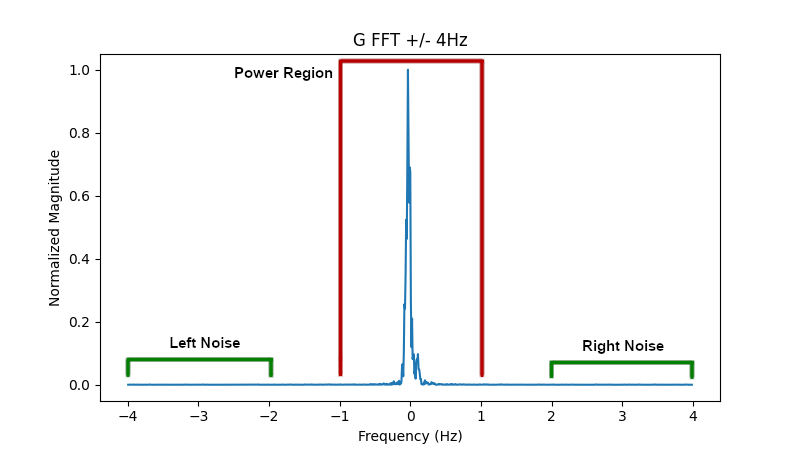It starts by looking in the power region for the maximum signal (as calculated as the sum of the squares of the real and imaginary components of the signal). This is stored as smax which is used to scale the whole FFT down between the values of 0 and 1.

In the noise regions an average signal on each side is calculated. The minimum value of the two regions is then subtracted from the FFT, effectively removing any offset above zero.

Pay close attention to how the loops check to see if i is negative and set j accordingly:

do i=-ia,ia
j=i
if(j.lt.0) j=i+nfft

For a negative i the FFT is actually indexed from the right hand side. Just visualizing the array without centering it will yield confusing results!

## Finding w50

To fully understand the next part of the algorithm, it helps to pay close attention to the name of the variable it returns: w50. A bit of googling yields this paper in which Joe Taylor defines w50

For practical purposes related to amateur EME communication, I define two parameters for measuring frequency spread: w50 and w10. Both are readily determined from autocorrelation functions of the echo spectra. Loosely speaking, they represent the full widths of those functions at the 50% (–3 dB) and 10% (–10 dB) points. In other words, w50 represents a range of frequencies containing half of the echo power, while w10 contains 90% of the power.
— Joe Taylor K1JT
Frequency-Dependent Characteristics of the EME Path

The total power for the power region is stored in sum1. The Fortran code loops through the power region again, this time summing up the power as it goes and storing the index when it reaches 25 percent, 50 percent, and 75 percent in xi1, xi2, and xi3 respectively:

ia=nint(1.0/df) + 1
sum2=0.0
xi1=-999
xi2=-999
xi3=-999
sum2z=0.
do i=-ia,ia                !Find freq range that has 50% of signal power
sum2=sum2 + ss(i)-avg
if(sum2.ge.0.25*sum1 .and. xi1.eq.-999.0) then
xi1=i - 1 + (sum2-0.25*sum1)/(sum2-sum2z)
endif
if(sum2.ge.0.50*sum1 .and. xi2.eq.-999.0) then
xi2=i - 1 + (sum2-0.50*sum1)/(sum2-sum2z)
endif
if(sum2.ge.0.75*sum1) then
xi3=i - 1 + (sum2-0.75*sum1)/(sum2-sum2z)
exit
endif
sum2z=sum2
enddo
xdiff=sqrt(1.0+(xi3-xi1)**2) !Keep small values from fluctuating too widely
fmid=xi2*df                  !Frequency midpoint of signal powere

You may also notice the indexes it stores aren’t technically integers. It performs an overshoot correction of the form:

$$OvershootCorrection = 1 + {(CurrentPower - ExpectedPower) \over (CurrentPower - PreviousPower)}$$

In this way if the percent power is somewhere between two indices, it can make a linear estimate as to where it would be and return a float.

The algorithm then takes the difference between the 75% power index and the 25% power index and attempts to prevent index differences less than one through the use of:

$$y=\sqrt{1+x^2}$$

Graphing this shows how the minimum xdiff will be one: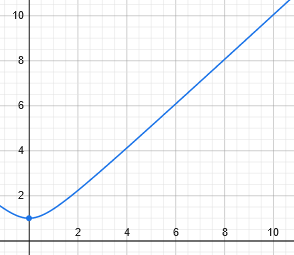The difference in indexes is then converted to a difference in frequencies and voilà, you have the spread of frequencies over which half of the power of the transmission is contained: w50.

## General Algorithm

To summarize, here are the basic steps of the algorithm for FSTW4 Doppler spread calculations:

1. Using the tones of the message construct an ideal complex waveform of what it should be scaled between -1 and 1.

2. Scale the received data to be between 1 and -1 and multiply it by the ideal waveform. This yields the estimated channel gain data, g, as discussed in the QEX paper.

3. Perform a forward, complex-to-complex FFT on the g at a resolution of twice the samples you started with.

4. Find the maximum power (real component squared plus imaginary component squared) around +/- 1 Hz of the center.

5. Scale by the maximum power so the FFT is between 0 and 1.

6. Find the average magnitude between -2 and -4 Hz as well as average magnitude between 2 and 4 Hz. This is the left and right noise.

7. Use the minimum noise and subtract it from the FFT.

8. Find the total power around the +/- 1 Hz region.

9. Iterate through the +/- 1 Hz region summing up the power to find the indices where you get 25% and 75% of the total magnitude (some steps can be taken to account for overshoot).

10. Take the difference of the indices ensuring that the minimum value is at least one one and convert those indexes to a frequency in Hz. This is w50.

## Python Implementation

I’d be remiss if I didn’t check to see that my understanding was complete by implementing the algorithm myself. What follows is a Python implementation, using the same data (including the ideal generated waveform) as the Fortran implementation.

import math
from numpy.fft import fft
import numpy as np
import matplotlib.pyplot as plt

RF_DATA_FILE = 'iwave.dat'
IDEAL_FILE = 'cwave.dat'
TIME = 60
SAMPLE_RATE = 12000
samples = TIME * SAMPLE_RATE
g_fft_size = samples * 2
index_per_freq = g_fft_size / SAMPLE_RATE
freq_per_index = 1 / index_per_freq
FOCUS_REGION = 4 # we really only care about frequencies +/- 4 Hz
POWER_REGION = 1 # we look for the maximum power to normalize in +/- 1 Hz
NOISE_REGION = 2 # we look for the noise 2 Hz in from outsides of the FOCUS region

# theoretically our RF data is between -32768 and 32768
# this scale factor makes it a float between -1 and 1
rf_scale_factor = 1.0 / 32768

rf_data = np.empty(samples, dtype=float)
with open(RF_DATA_FILE, 'r') as data_file:
i = 0
split_line = line.split()
rf_data[i] = int(split_line)
i += 1
if i > samples:
break
print(f"Read {rf_data.size} samples of RF data from {RF_DATA_FILE}")

ideal = np.empty(samples, dtype=complex)
with open(IDEAL_FILE, 'r') as data_file:
i = 0
split_line = line.split()
ideal[i] = complex(float(split_line), float(split_line))
i += 1
if i > samples:
break
print(f"Read {ideal.size} samples of the ideal waveform from {IDEAL_FILE}")

g = rf_scale_factor * rf_data * np.conj(ideal)
g_fft = fft(g, g_fft_size)

# grab the focus region, don't forget negative values wrap around
# we center the focus region in our array and from complex to just magnitude
di = int(index_per_freq * FOCUS_REGION)
g_fft_focus = np.empty(di * 2, dtype=float)
j = 0
for i in range(-1 * di, di):
if i < 0:
i += g_fft_size
g_fft_focus[j] = g_fft[i].real**2 + g_fft[i].imag**2
j += 1

# find the maximum power in the +/- POWER_REGION Hz region
# don't forget that the frequency has now shifted to start at -FOCUS_REGION Hz
power_start = int(index_per_freq * (FOCUS_REGION - POWER_REGION))
power_end = int(power_start + index_per_freq * (POWER_REGION * 2))
smax = g_fft_focus[power_start:power_end].max()
print(f"smax: {smax}")

# using smax, scale the FFT to be between 0 and 1
g_fft_focus /= smax

# calculate the noise average
left_noise = np.average(g_fft_focus[0:int(index_per_freq * NOISE_REGION)])
right_noise = np.average(g_fft_focus[:-int(index_per_freq*NOISE_REGION)])
noise = min(left_noise, right_noise)

# subtract out the noise
g_fft_focus -= noise

# calculate the power around POWER_REGION
total_power = np.sum(g_fft_focus[power_start:power_end])

# find the indexes around the power region where we hit 25% and 75% of the power
current_power = 0.0
p25_index = None
p75_index = None
for i in range(power_start, power_end):
current_power += g_fft_focus[i]
power_percent = current_power / total_power
if (power_percent >= 0.25) and p25_index == None:
p25_index = i
if power_percent >= 0.75:
p75_index = i
break
prev_power = current_power

xdiff = math.sqrt(1 + (p75_index - p25_index)**2)
w50 = xdiff * freq_per_index
print(f"Doppler Spread (w50): {w50}")

Running this gives me 0.051 for the Doppler spread, which is within 6% of the 0.054 the Fortran code gives.

## Conclusion

You can find the Python implementation, the data used, the scripts used to make the plots, and my butchered fst4_decode.f90 in this repo. Hopefully with a greater understanding of how this algorithm works, it can be implemented for WSPR signals. If you don’t want to dive into the C code that makes up wsprd, it may even be possible to do it based on the c2 files that wsprdaemon already analyzes. The main difference would probably be in setting FOCUS_REGION, POWER_REGION, and NOISE_REGION.

1. I found this doesn’t make a huge difference and I left it out of my Python implementation.